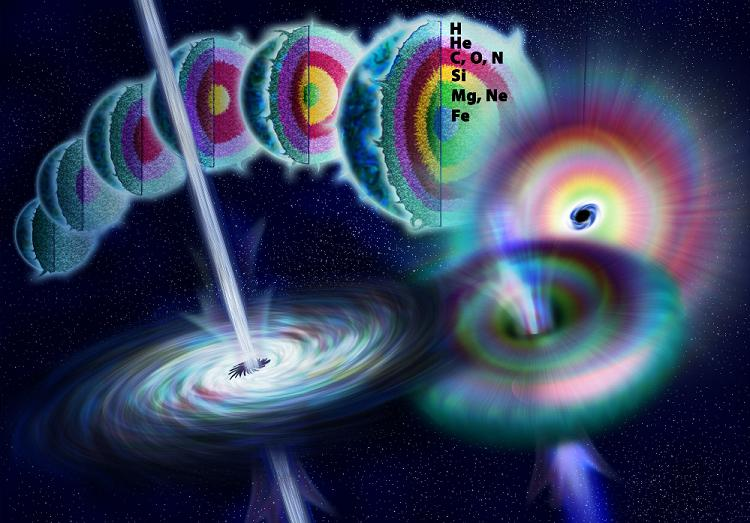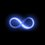This is an Applied Project from James Stewart's Calculus 6E - Instructor's Edition. It might take a long time, so don't get into it if you're in a hurry. Have fun.

Any object emits radiation when heated. A blackbody is a system that absorbs all the radiation that falls on it. For instance, a matte black surface or a large cavity with a small hole in its wall (like a blastfurnace) is a blackbody and emits blackbody radiation. Even the radiation from the sun is close to being a blackbody radiation.

Proposed in the late 19th century, the Rayleigh-Jeans Law expresses the energy density of blackbody radiation of wavelength $\lambda$ as

$f(\lambda)=\frac{8\pi kT}{\lambda^4}$

where $\lambda$ is measured in meters, $T$ is te temperature in kelvins $K$, and $k$ is Boltzmann's constant. The Rayleigh-Jeans Law agrees with experimental measures for long wavelengths but disagrees drastically for short wavelengths. [The law predicts that $f(\lambda)\rightarrow\infty$ as $\lambda \rightarrow 0\%+$ but experiments have shown that $f\lambda \rightarrow 0$.] This fact is known as the ultraviolet catastrophe.

In 1900 Max Planck found a better model (known now as Planck's Law) for blackbody radiation:

$f(\lambda)=\frac{8\pi hc\lambda^{-5}}{e^{\frac{hc}{(\lambda KT)}}-1}$

where $\lambda$ is measured in meters, $T$ is the temperature (in kelvins), and

$h=$ Planck's constant $=$$6.6262\times 10^{-34}J\cdot s$

$c=$ speed of light $=2.997925\times 10^8m/s$

$k=$ Boltzmann's constant $=1.3807\times 10^{-23}J/K$.

I. Use L'Hôpital's Rule to show that

$\lim _{ \lambda\rightarrow0^+ }{ f(\lambda) }=0$

for Planck's Law. So this law models blackbody radiation better than the Rayleigh-Jeans Law for short wavelengths.

II. Use a Taylor polynomial to show that, for large wavelengths, Planck's Law gives approximately the same values as the Rayleigh-Jeans Law.

III. Graph $f$ as given by both laws on the same screen and comment on the similarities and differences. Use $T=5700K$ (the temperature of the sun (on surface)). (You may want to change from meters to the more convenient unit of micrometers: $1\mu=10^{-6}m$).

IV. Use your graph in Problem 3 to estimate the value of $\lambda$ for which $f(\lambda)$ is a maximum under Plack's Law.

V. Investigate how the graph of $f$ changes as $T$ varies. (Use Planck's Law.) In particular, graph $f$ for the stars Betelgeuse ($T=3400K$), Procyon ($T=6400K$), and Sirius ($T=9200K$) as well as the sun. How does the toal radiation emitted (the area under the curve) var with $T$? Use the graph to comment on why Sirius is known as a blue star and Betelgeuse as a red star.

Problem credit: James Stewart: _Calculus: _6th Edition, Instructor's Edition.Note by John M.
6 years, 11 months ago

This discussion board is a place to discuss our Daily Challenges and the math and science related to those challenges. Explanations are more than just a solution — they should explain the steps and thinking strategies that you used to obtain the solution. Comments should further the discussion of math and science.

When posting on Brilliant:

• Use the emojis to react to an explanation, whether you're congratulating a job well done , or just really confused .
• Ask specific questions about the challenge or the steps in somebody's explanation. Well-posed questions can add a lot to the discussion, but posting "I don't understand!" doesn't help anyone.
• Try to contribute something new to the discussion, whether it is an extension, generalization or other idea related to the challenge.

MarkdownAppears as
*italics* or _italics_ italics
**bold** or __bold__ bold
- bulleted- list
• bulleted
• list
1. numbered2. list
1. numbered
2. list
Note: you must add a full line of space before and after lists for them to show up correctly
paragraph 1paragraph 2

paragraph 1

paragraph 2

[example link](https://brilliant.org)example link
> This is a quote
This is a quote
    # I indented these lines
# 4 spaces, and now they show
# up as a code block.

print "hello world"
# I indented these lines
# 4 spaces, and now they show
# up as a code block.

print "hello world"
MathAppears as
Remember to wrap math in $$ ... $$ or $ ... $ to ensure proper formatting.
2 \times 3 $2 \times 3$
2^{34} $2^{34}$
a_{i-1} $a_{i-1}$
\frac{2}{3} $\frac{2}{3}$
\sqrt{2} $\sqrt{2}$
\sum_{i=1}^3 $\sum_{i=1}^3$
\sin \theta $\sin \theta$
\boxed{123} $\boxed{123}$